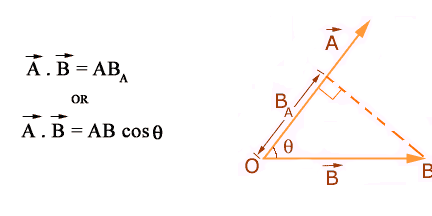# Commutative Law For Dot Product

Commutative law for dot product
This law states that:
“The scalar product of two vectors A and B is equal to the magnitude of vector A times the projection of B onto the direction of vector A."
Consider two vectors A and B, the angle between them is q.This shows that the dot product of two vectors does not change with the change in the order of the vectors to be multiplied.
This fact is known as the commutative of dot product.